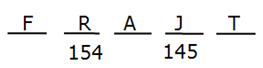# IBPS / LIC Clerk Prelims Reasoning Ability Questions 2019 (Day-03)

Dear Aspirants, Our IBPS Guide team is providing new series of Reasoning Questions for IBPS Clerk Prelims 2019 so the aspirants can practice it on a daily basis. These questions are framed by our skilled experts after understanding your needs thoroughly. Aspirants can practice these new series questions daily to familiarize with the exact exam pattern and make your preparation effective.

Check here for IBPS Clerk Prelims Mock Test 2019

##### Check here for IBPS Clerk  Mock Test 2019

[WpProQuiz 7226]

Seating arrangement

Direction (1-5): Read the following information carefully and answer the questions given below.

There are eight persons P, H, Y, I, J, D, Q and W sitting in a row facing north but not necessarily in the same order.

Y sits fourth to the left of D. Y does not sits any extreme of the row. I sits third to the left of Q.  J and W are immediate neighbors. Number of persons between H and W is same as the number of persons between I and Q. I is not the immediate neighbor of Y. H sits left of P. Number of persons sits between Y and W is one more than the number of persons between Y and J. Number of persons between H and W is same as the number of persons between I and J.

1)  Who among the following are immediate neighbors of Y?

a) I, H

b) J, H

c) J, I

d) H, W

e) None of these.

2) Who sits second to the left of I?

a) Y

b) J

c) D

d) Q

e) None of these.

3) How many persons are sits between D and H?

a) 0

b) 1

c) 2

d) 3

e) None of these.

4) Find the odd one out of the rest?

a) I

b) Y

c) D

d) J

e) Q

5) Which of the following is true according to P?

a) I sits immediate left of P.

b) Only two persons sits between P and Y.

c) Only one person sits between P and Q

d) All are true.

e) None is true.

Direction Sense

Direction (6-8): Read the following information carefully and answer the questions given below.

A man walks from Point A to Point B. He walks from point A walks 8m south, then takes left walks 5m again takes left walks 4m. Then takes right walks 3m and then take left walks 4mto reach point B.

6) What is the shortest distance from Point A to Point B?

a) 7m

b) 8m

c) 9m

d) 10m

e) None of these.

7) Point A is in which direction with respect to point B?

a) East

b) North

c) South

d) West

e) None of these.

8) What is the total distance travelled by the man?

a) 20

b) 21

c) 22

d) 23

e) None of these.

Order and Ranking

Direction (9-10): Read the following information carefully and answer the questions given below.

There are five persons A, T, R, J and F standing in a row arranged descending order according to their height.

R is taller than J but shorter than F. Only two persons are shorter than A. J is not the shortest person among them. The one who is second shortest person height is 145 cm.  The one who is second tallest person height is 154 cm.

9) What is the possible height of F?

a) 153 cm

b) 140 cm

c) 160 cm

d) 151 cm

e) None of these.

10) Who is the tallest person?

a) R

b) F

c) A

d) J

e) None of these

Directions (1-5):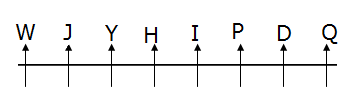Y sits fourth to the left of D. Y does not sits any extreme of the row.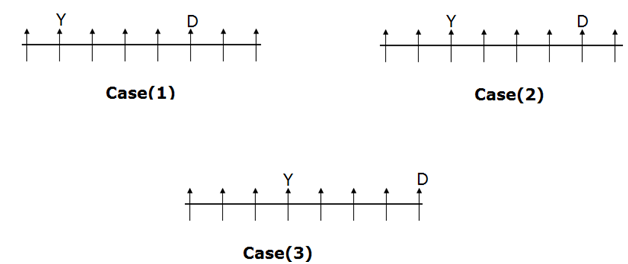I sits third to the left of Q.  I is not the immediate neighbor of Y.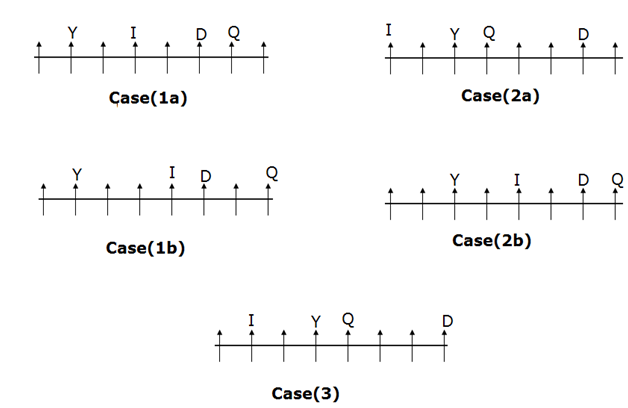J and W are immediate neighbors. H sits left of P.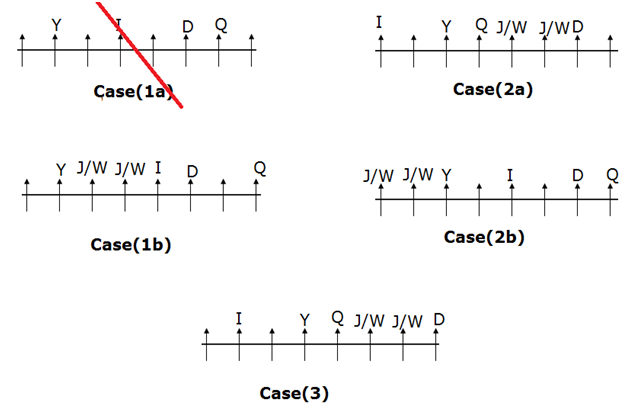Case (1a) gets eliminated because we have no place to fix the position of J and W.

H sits left of P.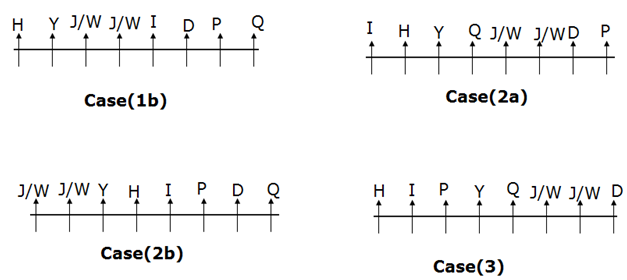Number of persons sits between Y and W is one more than the number of persons between Y and J.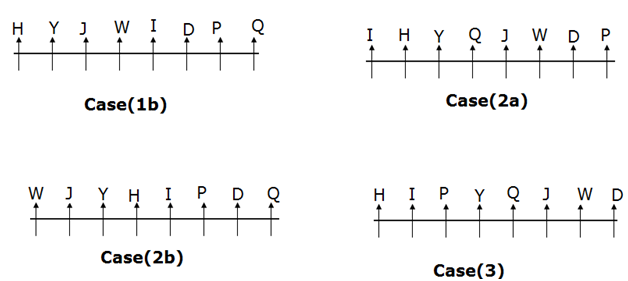Number of persons between H and W is same as the number of persons between I and J.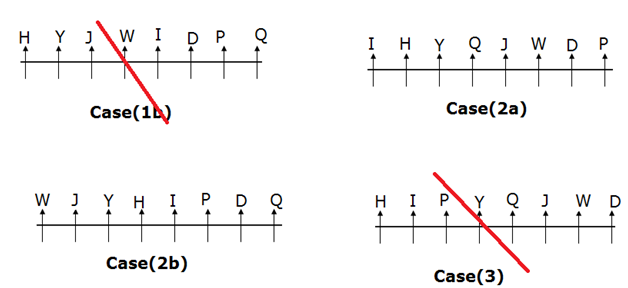Case (1b) and Case (3) gets eliminated because Number of persons between H and W is same as the number of persons between I and J.

Number of persons between H and W is same as the number of persons between I and Q.Case (2a) gets eliminated because Number of persons between H and W is same as the number of persons between I and Q.

Hence Case (2b) will be the final arrangement.

Directions (6-8) :

5+3=8

Point A is west of Point B.

8+5+4+3+4=24.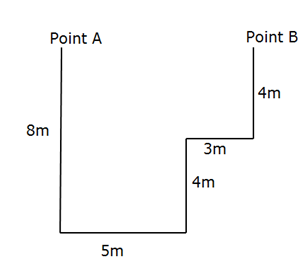Directions (9-10) :